# AI：拿来主义——预训练网络（一）

2020/03/02 10:14

2005 年左右，李飞飞结束了他的博士生涯，开始了他的学术研究不就她就意识到了一个问题，在此之前，人们都尽可能优化算法，认为无论数据如何，只要算法够好，就能做出更好的决策，李飞飞意识到了这个问题的局限性，恰巧她还是一个行动派，她要做出一个无比庞大的数据集，尽可能描述世界上一切物体的数据集，下载图片，给没一张图片做标注，简单而无聊，当然后来这项工作放到了亚马逊的众包平台上，全世界无数的人参与了这个伟大的项目，到此刻为止，已经有 14,197,122 张图片（一千四百万张），21841 个分类。在这个发展的过程中，人们也发现了这个数据集带来的成功远比预想的要多，甚至现在被认为最有前景的深度卷积神经网络的提出也与 ImageNet 不无关系。我忘记了谁这么说过：“就单单这一个数据集，就可以让李飞飞数据科学这个领域拥有一席之地”。暂且不说这么说是否准确，但这个数据集仍然在创造新的突破。（我曾经在台下听过李飞飞一次演讲，现在想想还觉得甚是激动，她真的充满热情）。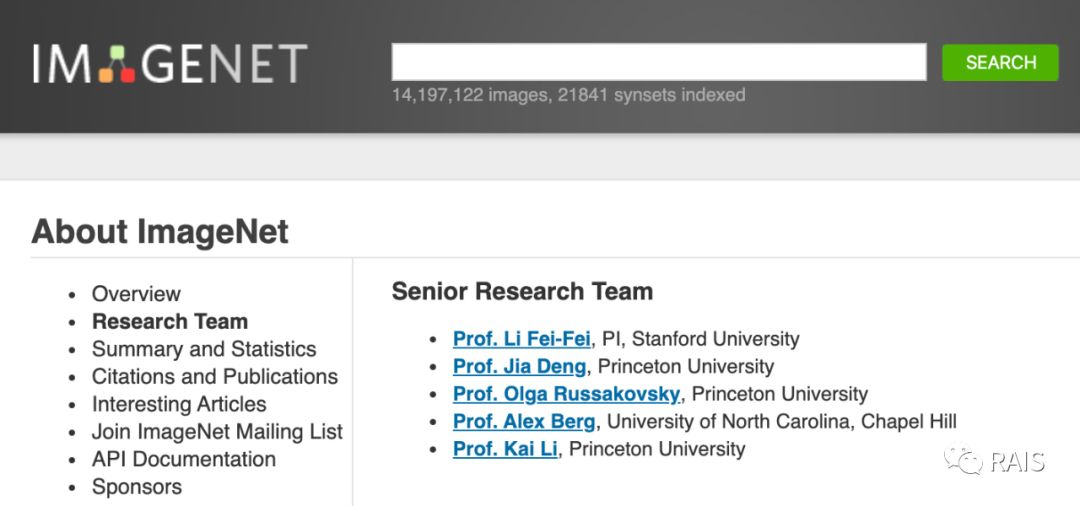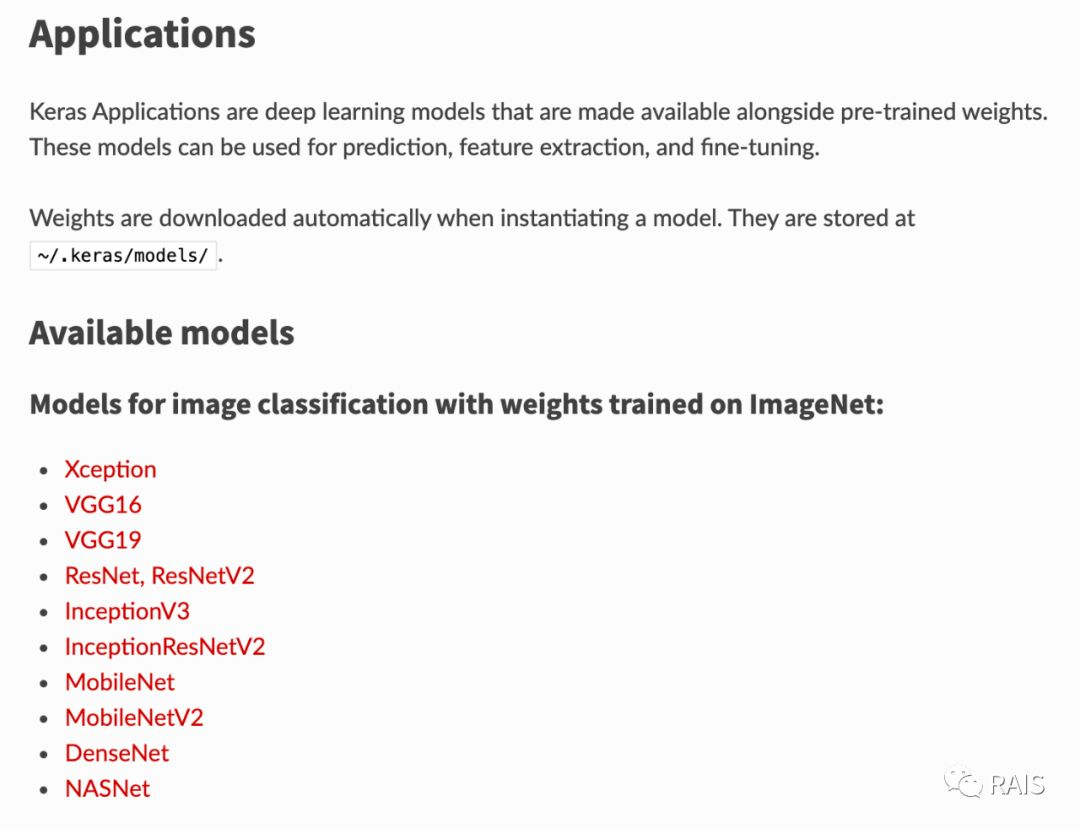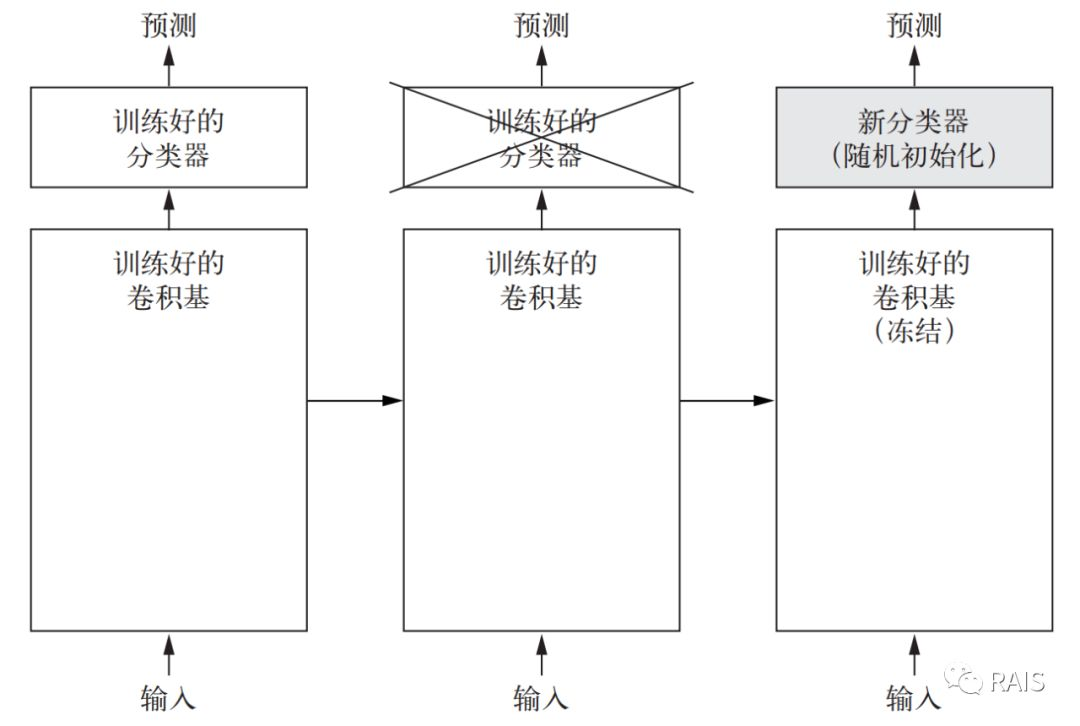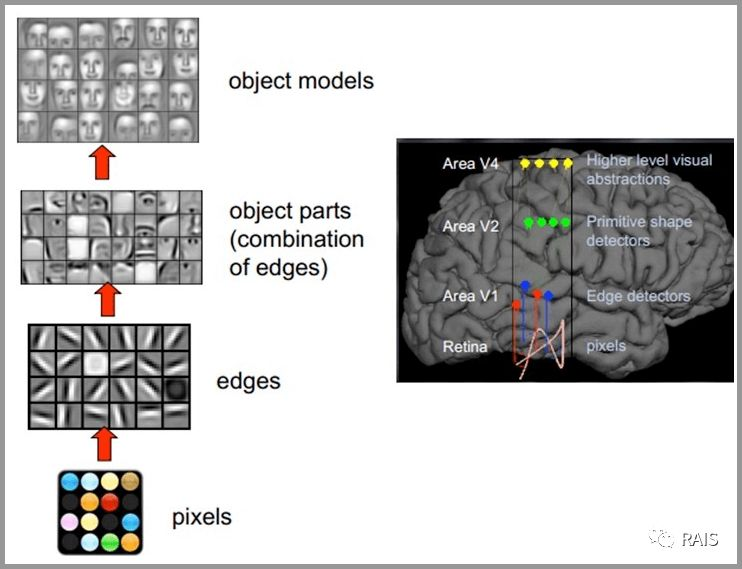https://github.com/fchollet/deep-learning-models/releases


#!/usr/bin/env python3
​
import os
import time
​
import matplotlib.pyplot as plt
import numpy as np
from keras import layers
from keras import models
from keras import optimizers
from keras.applications import VGG16
from keras.preprocessing.image import ImageDataGenerator
​
​
def extract_features(directory, sample_count):
# 图片转换区间
datagen = ImageDataGenerator(rescale=1. / 255)
batch_size = 20
conv_base = VGG16(weights='imagenet',
include_top=False,
input_shape=(150, 150, 3))
​
conv_base.summary()
​
features = np.zeros(shape=(sample_count, 4, 4, 512))
labels = np.zeros(shape=(sample_count))
# 读出图片，处理成神经网络需要的数据格式，上一篇文章中有介绍
generator = datagen.flow_from_directory(
directory,
target_size=(150, 150),
batch_size=batch_size,
class_mode='binary')
i = 0
for inputs_batch, labels_batch in generator:
print(i, '/', len(generator))
# 提取特征
features_batch = conv_base.predict(inputs_batch)
features[i * batch_size: (i + 1) * batch_size] = features_batch
labels[i * batch_size: (i + 1) * batch_size] = labels_batch
i += 1
if i * batch_size &gt;= sample_count:
break
​
# 特征和标签
return features, labels
​
​
def cat():
base_dir = '/Users/renyuzhuo/Desktop/cat/dogs-vs-cats-small'
train_dir = os.path.join(base_dir, 'train')
validation_dir = os.path.join(base_dir, 'validation')
​
# 提取出的特征
train_features, train_labels = extract_features(train_dir, 2000)
validation_features, validation_labels = extract_features(validation_dir, 1000)
​
# 对特征进行变形展平
train_features = np.reshape(train_features, (2000, 4 * 4 * 512))
validation_features = np.reshape(validation_features, (1000, 4 * 4 * 512))
​
# 定义密集连接分类器
model = models.Sequential()
model.add(layers.Dense(256, activation='relu', input_dim=4 * 4 * 512))
​
# 对模型进行配置
model.compile(optimizer=optimizers.RMSprop(lr=2e-5),
loss='binary_crossentropy',
metrics=['acc'])
​
# 对模型进行训练
history = model.fit(train_features, train_labels,
epochs=30,
batch_size=20,
validation_data=(validation_features, validation_labels))
​
# 画图
acc = history.history['acc']
val_acc = history.history['val_acc']
loss = history.history['loss']
val_loss = history.history['val_loss']
epochs = range(1, len(acc) + 1)
plt.plot(epochs, acc, 'bo', label='Training acc')
plt.plot(epochs, val_acc, 'b', label='Validation acc')
plt.title('Training and validation accuracy')
plt.legend()
plt.show()
plt.figure()
plt.plot(epochs, loss, 'bo', label='Training loss')
plt.plot(epochs, val_loss, 'b', label='Validation loss')
plt.title('Training and validation loss')
plt.legend()
plt.show()
​
​
if __name__ == "__main__":
time_start = time.time()
cat()
time_end = time.time()
print('Time Used: ', time_end - time_start)​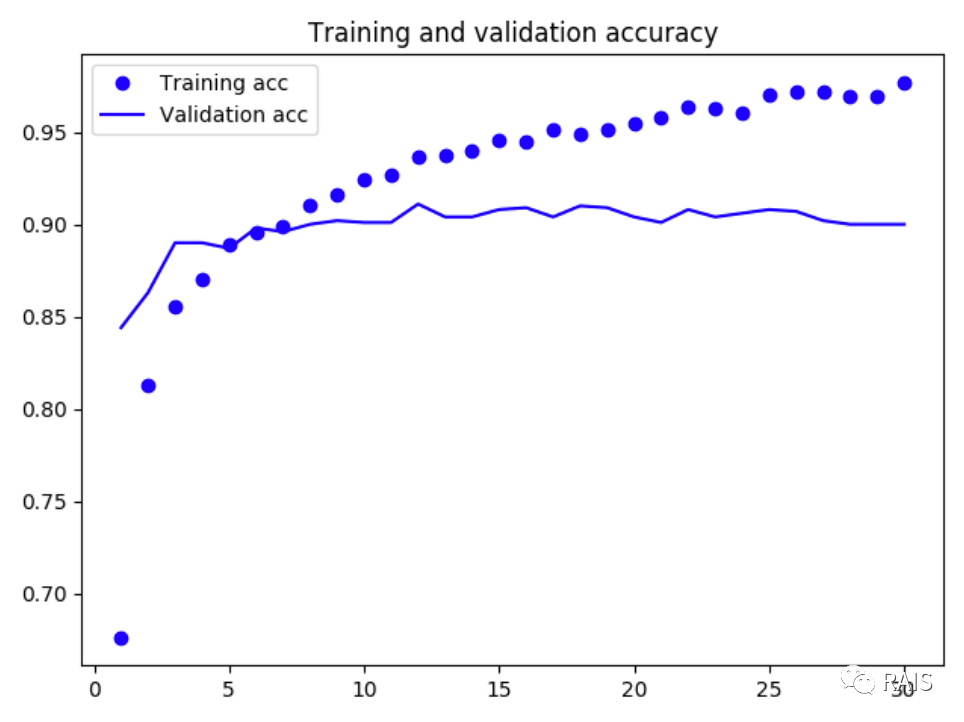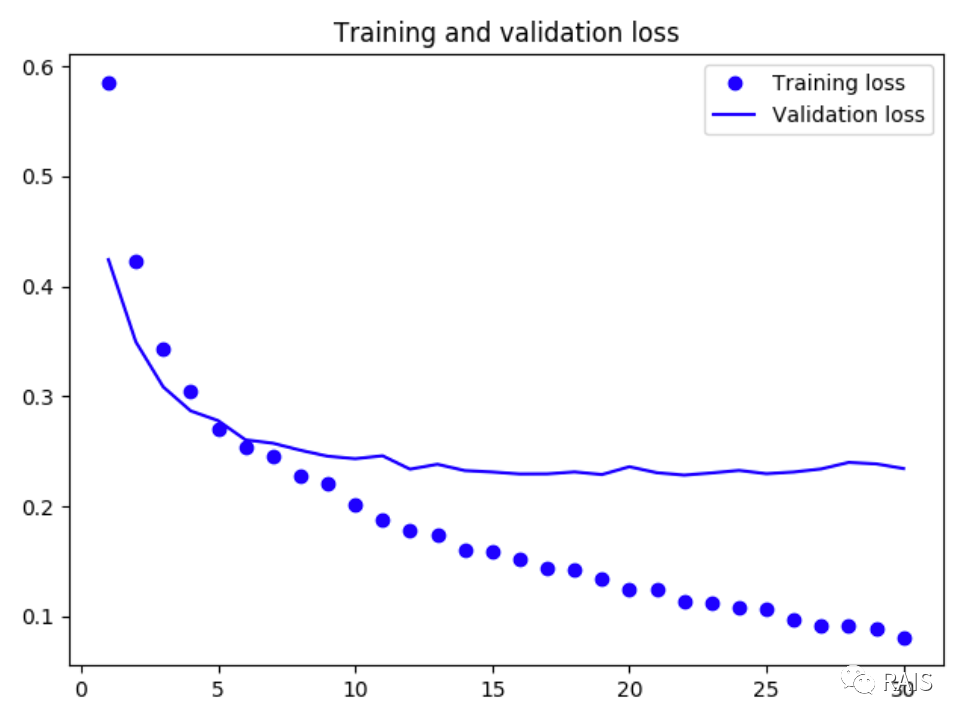conv_base.trainable = False
​
model = models.Sequential()


> 首发自公众号：RAIS

0
3 收藏

### 作者的其它热门文章2020/03/02 19:54

2020/03/02 23:11

3 评论
3 收藏
0